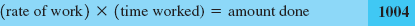### 3.7. Applications to Work, Fluid Flow, and Energy Flow

#### 3.7.1. Solving Rate Problems

We already considered word problems involving constant rates when we learned about uniform motion. This will now make it easier for us to solve other problems in which the quantities are related in the same way. These are work, fluid flow, and energy flow.

 Uniform motion: amount traveled = rate of travel × time traveled Work: amount of work done = rate of work × time worked Fluid flow: amount of flow = flow rate × duration of flow Energy flow: amount of energy = rate of energy flow × time

Notice that these equations are mathematically identical. Many applications involving motion, flow of fluids or solids, flow of heat, electricity, solar energy, mechanical energy, and so on, can be handled in the same way.

To convince yourself of the similarity of all of these types of problems, consider the following work problem:

If worker M can do a certain job in 5 h, and N can do the same amount in 8 h, how long will it take M and N together to do that same amount?

Are the following problems really any different?#### 3.7.2. Work

To tackle work problems, we need one simple idea.

NOTEHere we require that the rate of work is constant. We often use this equation to find the rate of work for a person or a machine.

Get Technical Mathematics, Sixth Edition now with the O’Reilly learning platform.

O’Reilly members experience live online training, plus books, videos, and digital content from nearly 200 publishers.